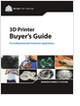MaterialsDesign CenterProcessesUnit ConversionFormulasMathematicsCalculatorsDiscussion ForumTrade PublicationsDirectory Service
Selecting the Right 3D Printer

Discover how to choose the right 3D printer for your needs and the key performance attributes to consider.

CNC Machining Design Guide

3D Scanners

A white paper to assist in the evaluation of 3D scanning hardware solutions.

Essentials of Manufacturing

Information, coverage of important developments and expert commentary in manufacturing.

more free publicationsGlossary » Units » Power (Heat Flow) » Calorie (IT) Per MinuteCalorie (IT) Per Minute (cal (IT)/min) is a unit in the category of Power (Heat flow). It is also known as calorie per minute, calories per minute, cal/minute, calorie/minute. Calorie (IT) Per Minute (cal (IT)/min) has a dimension of ML2T-3 where M is mass, L is length, and T is time. It can be converted to the corresponding standard SI unit W by multiplying its value by a factor of 0.06977899999.
Note that the seven base dimensions are M (Mass), L (Length), T (Time), Q (Temperature), N (Aamount of Substance), I (Electric Current), and J (Luminous Intensity).

Other units in the category of Power (Heat flow) include Abwatt (emu of Power) (aW), British Thermal Unit (IT) Per Hour (Btu (IT)/h), British Thermal Unit (IT) Per Minute (Btu (IT)/min), British Thermal Unit (IT) Per Second (Btu (IT)/s), British Thermal Unit (therm.) Per Hour (Btu (therm.)/h), British Thermal Unit (therm.) Per Minute (Btu (therm.)/min), British Thermal Unit (therm.) Per Second (Btu (therm.)/s), Calorie (IT) Per Hour (cal (IT)/h), Calorie (IT) Per Second (cal (IT)/s), Calorie (therm.) Per Hour (cal (therm.)/h), Calorie (therm.) Per Minute (cal (therm.)/min), Calorie (therm.) Per Second (cal (therm.)/s), Cheval-Vapeur (horsepower) (cv, HP), Dyne-Centimeter Per Second (dyn-cm/s), Erg Per Second (erg/s), Foot-Pound Force Per Hour (ft-lbf/h), Foot-Pound Force Per Minute (ft-lbf/min), Foot-Pound Force Per Second (ft-lbf/s), Foot-Poundal Per Second (ft-pdl/s), Horsepower (550 Ft-Lbf/s) (HP), Horsepower (boiler) (HP), Horsepower (British) (BHP, hp), Horsepower (cheval-Vapeur) (cv, HP), Horsepower (electric) (HP), Horsepower (metric) (HP), Horsepower (water) (HP), Kilogram Force-Meter Per Second (kgf-m/s), Kilowatt (kW), Prony, Ton (refrigeration, UK) (CTR (UK)), Ton (refrigeration, US) (CTR (US)), Volt-Ampere (VA), Watt (W), Watt (int. Mean) (W), and Watt (int. US) (W).N/ARelated PagesSelecting the Right 3D Printer

Discover how to choose the right 3D printer for your needs and the key performance attributes to consider.CNC Machining Design Guide